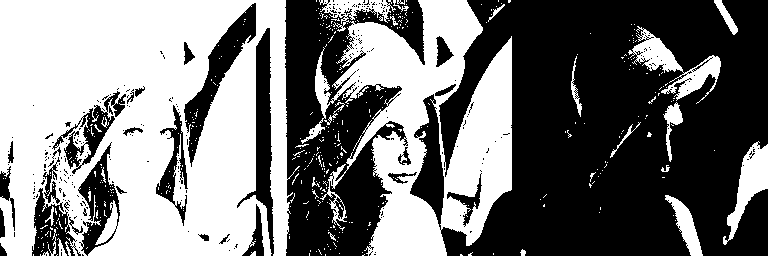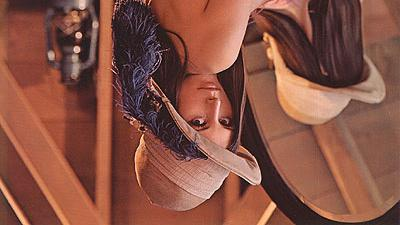# Image processing with Python, NumPy

Posted: 2019-05-14 / Modified: 2020-10-20 / Tags: Python, NumPy, Image Processing

By reading the image as a NumPy array `ndarray`, various image processing can be performed using NumPy functions.

By the operation of ndarray, you can get and set (change) pixel values, trim images, concatenate images, etc. Those who are familiar with NumPy can do various image processing without using libraries such as OpenCV.

Even when using OpenCV, OpenCV for Python treats image data as `ndarray`, so it is useful to know how to use NumPy (`ndarray`). In addition to OpenCV, there are many libraries such as scikit-image that treat images as `ndarray`.

• How to read image file as NumPy array `ndarray`
• How to save NumPy array `ndarray` as image file

Examples of image processing with NumPy (`ndarray`):

• Get and set (change) pixel values
• Generation of single color image and concatenation
• Negative / positive inversion (inversion of pixel value)
• Color reduction
• Binarization
• Gamma correction
• Trimming with slice
• Split with slice or function
• Paste with slice
• Rotate and flip

Sample codes on this article use Pillow to read and save image files. If you want to use OpenCV, see the following article.

See also the following article about Pillow. Simple operations such as reading and saving images, resizing and rotating images can be done by Pillow alone.

## How to read an image file as ndarray

Take the following image as an example.Passing the image data read by `PIL.Image.open()` to `np.array()` returns 3D `ndarray` whose shape is `(row (height), column (width), color (channel))`.

``````from PIL import Image
import numpy as np

im = np.array(Image.open('data/src/lena.jpg'))

print(type(im))
# <class 'numpy.ndarray'>

print(im.dtype)
# uint8

print(im.shape)
# (225, 400, 3)
``````

The order of colors (channels) is RGB (red, green, blue). Note that it is different from the case of reading with `cv2.imread()` of OpenCV.

If you convert the image to grayscale with `convert('L')` and then pass it to `np.array()`, it returns 2D `ndarray` whose shape is `(row (height), column (width))`.

``````im_gray = np.array(Image.open('data/src/lena.jpg').convert('L'))

print(im_gray.shape)
# (225, 400)
``````

You can also get `ndarray` from `PIL.Image` with `np.asarray()`. `np.array()` returns a rewritable `ndarray`, while `np.asarray()` returns a non-rewritable`ndarray`.

For `np.array()`, you can change the value of the element (pixel).

``````print(im.flags.writeable)
# True

print(im[0, 0, 0])
# 109

im[0, 0, 0] = 0

print(im[0, 0, 0])
# 0
``````

For `np.asarray()`, you cannot change value because rewriting is prohibited. It is possible to create a new `ndarray` based on the read `ndarray`.

``````im_as = np.asarray(Image.open('data/src/lena.jpg'))

print(type(im_as))
# <class 'numpy.ndarray'>

print(im_as.flags.writeable)
# False

# im_as[0, 0, 0] = 0
# ValueError: assignment destination is read-only
``````

The data type `dtype` of the read `ndarray` is `uint8` (8-bit unsigned integer).

If you want to process it as a floating point number `float`, you can convert it with `astype()` or specify the data type in the second argument of `np.array()` and `np.asarray()`.

``````im_f = im.astype(np.float64)
print(im_f.dtype)
# float64

im_f = np.array(Image.open('data/src/lena.jpg'), np.float64)
print(im_f.dtype)
# float64
``````

See the following article for more information about the data type `dtype` in NumPy.

## How to save NumPy array ndarray as image file

Passing `ndarray` to `Image.fromarray()` returns `PIL.Image`. It can be saved as an image file with `save()` method. The format of the saved file is automatically determined from the extension of the path passed in the argument of `save()`.

``````pil_img = Image.fromarray(im)
print(pil_img.mode)
# RGB

pil_img.save('data/temp/lena_save_pillow.jpg')
``````

A grayscale image (2D array) can also be passed to `Image.fromarray()`. `mode` automatically becomes `'L'` (grayscale). It can be saved with `save()`.

``````pil_img_gray = Image.fromarray(im_gray)
print(pil_img_gray.mode)
# L

pil_img_gray.save('data/temp/lena_save_pillow_gray.jpg')
``````

If you just want to save it, you can write it in one line.

``````Image.fromarray(im).save('data/temp/lena_save_pillow.jpg')
Image.fromarray(im_gray).save('data/temp/lena_save_pillow_gray.jpg')
``````

If the data type `dtype` of `ndarray` is `float`, etc., an error will occur, so it is necessary to convert to `uint8`.

``````# pil_img = Image.fromarray(im_f)
# TypeError: Cannot handle this data type

pil_img = Image.fromarray(im_f.astype(np.uint8))
pil_img.save('data/temp/lena_save_pillow.jpg')
``````

Note that if the pixel value is represented by `0.0` to `1.0`, it is necessary to multiply by `255` and convert to `uint8` and save.

With `save()`, parameters according to the format can be passed as arguments. See Image file format for details.

For example, in the case of JPG, you can pass the quality of the image to the argument `quality`. It ranges from `1` (the lowest) to `95` (the highest) and defaults to `75`.

## Get and set (change) pixel values

You can get the value of a pixel by specifying the coordinates at the index `[row, columns]` of `ndarray`. Note that the order is `y, x` in xy coordinates. The origin is the upper left.

``````from PIL import Image
import numpy as np

im = np.array(Image.open('data/src/lena.jpg'))

print(im.shape)
# (225, 400, 3)

print(im[100, 150])
# [111  81 109]

print(type(im[100, 150]))
# <class 'numpy.ndarray'>
``````

The above example shows the value at `(y, x) = (100, 150)`, i.e. the 100th row and 150th column of pixels. As mentioned above, the colors of the `ndarray` obtained using Pillow are in RGB order, so the result is `(R, G, B) = (111, 81, 109)`.

You can also use unpack to assign them to separate variables.

``````R, G, B = im[100, 150]

print(R)
# 111

print(G)
# 81

print(B)
# 109
``````

It is also possible to get the value by specifying the color.

``````print(im[100, 150, 0])
# 111

print(im[100, 150, 1])
# 81

print(im[100, 150, 2])
# 109
``````

You can also change to a new value. You can change RGB all at once, or you can change it with just a single color.

``````im[100, 150] = (0, 50, 100)

print(im[100, 150])
# [  0  50 100]

im[100, 150, 0] = 150

print(im[100, 150])
# [150  50 100]
``````

## Generation of single color image and concatenation

Generate single-color images by setting other color values to `0`, and concatenate them horizontally with `np.concatenate()`. You can also concatenate images using `np.hstack()` or `np.c_[]`

``````from PIL import Image
import numpy as np

im = np.array(Image.open('data/src/lena_square.png'))

im_R = im.copy()
im_R[:, :, (1, 2)] = 0
im_G = im.copy()
im_G[:, :, (0, 2)] = 0
im_B = im.copy()
im_B[:, :, (0, 1)] = 0

im_RGB = np.concatenate((im_R, im_G, im_B), axis=1)
# im_RGB = np.hstack((im_R, im_G, im_B))
# im_RGB = np.c_['1', im_R, im_G, im_B]

pil_img = Image.fromarray(im_RGB)
pil_img.save('data/dst/lena_numpy_split_color.jpg')
``````## Negative / positive inversion (invert pixel value)

It is also easy to calculate and manipulate pixel values.

A negative-positive inverted image can be generated by subtracting the pixel value from the max value (`255` for `uint8`).

``````import numpy as np
from PIL import Image

im = np.array(Image.open('data/src/lena_square.png').resize((256, 256)))

im_i = 255 - im

Image.fromarray(im_i).save('data/dst/lena_numpy_inverse.jpg')
``````Because the original size is too large, it is resized with `resize()` for convenience. The same applies to the following examples.

## Color reduction

Cut off the remainder of the division using `//` and multiply again, the pixel values become discrete and the number of colors can be reduced.

``````import numpy as np
from PIL import Image

im = np.array(Image.open('data/src/lena_square.png').resize((256, 256)))

im_32 = im // 32 * 32
im_128 = im // 128 * 128

im_dec = np.concatenate((im, im_32, im_128), axis=1)

Image.fromarray(im_dec).save('data/dst/lena_numpy_dec_color.png')
``````## Binarization

It is also possible to assign to black and white according to the threshold.

See the following articles for details.## Gamma correction

You can do anything you want with pixel values, such as multiplication, division, exponentiation, etc.

You don't need to use the for loop because the entire image can be calculated as it is.

``````from PIL import Image
import numpy as np

im = np.array(Image.open('data/src/lena_square.png'))

im_1_22 = 255.0 * (im / 255.0)**(1 / 2.2)
im_22 = 255.0 * (im / 255.0)**2.2

im_gamma = np.concatenate((im_1_22, im, im_22), axis=1)

pil_img = Image.fromarray(np.uint8(im_gamma))
pil_img.save('data/dst/lena_numpy_gamma.jpg')
``````As a result of the calculation, the data type `dtype` of `numpy.ndarray` is converted to the floating point number `float`. Note that you need to convert it to `uint8` when you save it.

## Trimming with slice

By specifying an area with slice, you can trim it to a rectangle.

``````from PIL import Image
import numpy as np

im = np.array(Image.open('data/src/lena_square.png'))

print(im.shape)
# (512, 512, 3)

im_trim1 = im[128:384, 128:384]
print(im_trim1.shape)
# (256, 256, 3)

Image.fromarray(im_trim1).save('data/dst/lena_numpy_trim.jpg')
``````See the following article for more information on slicing for `numpy.ndarray`.

It may be convenient to define a function that specifies the upper left coordinates and the width and height of the area to be trimmed.

``````def trim(array, x, y, width, height):
return array[y:y + height, x:x+width]

im_trim2 = trim(im, 128, 192, 256, 128)
print(im_trim2.shape)
# (128, 256, 3)

Image.fromarray(im_trim2).save('data/dst/lena_numpy_trim2.jpg')
``````If you specify outside the size of the image, it will be ignored.

``````im_trim3 = trim(im, 128, 192, 512, 128)
print(im_trim3.shape)
# (128, 384, 3)

Image.fromarray(im_trim3).save('data/dst/lena_numpy_trim3.jpg')
``````## Split with slice or function

You can also split the image by slicing.

``````from PIL import Image
import numpy as np

im = np.array(Image.open('data/src/lena_square.png').resize((256, 256)))

print(im.shape)
# (256, 256, 3)

im_0 = im[:, :100]
im_1 = im[:, 100:]

print(im_0.shape)
# (256, 100, 3)

print(im_1.shape)
# (256, 156, 3)

Image.fromarray(im_0).save('data/dst/lena_numpy_split_0.jpg')
Image.fromarray(im_1).save('data/dst/lena_numpy_split_1.jpg')
``````It is also possible to split the image with NumPy function.

`np.hsplit()` splits `ndarray` horizontally. If an integer value is specified for the second argument, `ndarray` is splitted equally.

``````im_0, im_1 = np.hsplit(im, 2)

print(im_0.shape)
# (256, 128, 3)

print(im_1.shape)
# (256, 128, 3)
``````

If a list is specified as the second argument, `ndarray` is splitted at the position of that values.

``````im_0, im_1, im_2 = np.hsplit(im, [100, 150])

print(im_0.shape)
# (256, 100, 3)

print(im_1.shape)
# (256, 50, 3)

print(im_2.shape)
# (256, 106, 3)
``````

`np.vsplit()` splits `ndarray` vertically. The usage of `np.vsplit()` is the same as `np.hsplit()`.

When an integer value is specified as the second argument with `np.hsplit()` or `np.vsplit()`, an error will occur if it cannot be splitted equally. `np.array_split()` adjusts the size appropriately and splits it.

``````# im_0, im_1, im_2 = np.hsplit(im, 3)
# ValueError: array split does not result in an equal division

im_0, im_1, im_2 = np.array_split(im, 3, axis=1)

print(im_0.shape)
# (256, 86, 3)

print(im_1.shape)
# (256, 85, 3)

print(im_2.shape)
# (256, 85, 3)
``````

## Paste with slice

Using slices, one array rectangle can be replaced with another array rectangle.

By using this, a part of the image or the entire image can be pasted to another image.

``````import numpy as np
from PIL import Image

src = np.array(Image.open('data/src/lena_square.png').resize((128, 128)))
dst = np.array(Image.open('data/src/lena_square.png').resize((256, 256))) // 4

dst_copy = dst.copy()
dst_copy[64:128, 128:192] = src[32:96, 32:96]

Image.fromarray(dst_copy).save('data/dst/lena_numpy_paste.jpg')
````````````dst_copy = dst.copy()
dst_copy[64:192, 64:192] = src

Image.fromarray(dst_copy).save('data/dst/lena_numpy_paste_all.jpg')
``````Note that an error will occur if the size of the area specified on the left side differs from the size of the area specified on the right side.

By the operation for each element (= pixel) of the array, two images can be alpha-blended or composited based on a mask image. See the following articles for details.## Rotate and flip

There are also functions that rotate the array and flip it up, down, left and right.

Original image:Rotated image:Flipped image: